# Texas Go Math Grade 5 Lesson 3.2 Answer Key Multiply Decimals and Whole Numbers

Refer to our Texas Go Math Grade 5 Answer Key Pdf to score good marks in the exams. Test yourself by practicing the problems from Texas Go Math Grade 5 Lesson 3.1 Answer Key Multiplication Patterns with Decimals.

## Texas Go Math Grade 5 Lesson 3.2 Answer Key Multiply Decimals and Whole Numbers

Investigate

Materials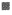decimal modelscolor pencils

Giant tortoises move very slowly. They can travel a distance of about 0.17 mile in 1 hour,
If a giant tortoise travels at the same speed, how far could it move in 4 hours?
A. Complete the statement to describe the problem.
I need to find how many total miles are in _0.17__ groups of _4__
• Write an expression to represent the problem. _0.17 X 4___

B. Use the decimal model to find the answer.
• What does each small square in the decimal model represent?

C. Shade a group of ___ squares to represent the distance a giant tortoise can move in 1 hour.

group of ___ squares until you have __ group of __ squares.

E. Record the total number of squares shaded. ___ squares
So, the giant tortoise can move ___ mile in 4 hours.

A. Total number of miles are in 0.17 groups of 4,
Wrote an expression to represent the problem 0.17 X 4,
B. Used the decimal model to find the answer,
Used small square in the decimal model represents,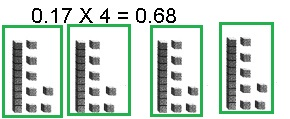C. Shaded a group of 4 squares to represent the distance a
giant tortoise can move in 1 hour.
group of __0.17_ squares until you have _4_ group of _0.17_ squares.

E. Record the total number of squares shaded. __0.68_ squares
So, the giant tortoise can move _0.68__ mile in 4 hours.

Explanation:
A. Total number of miles are in 0.17 groups of 4,
Wrote an expression to represent the problem 0.17 X 4,
B. Used the decimal model to find the answer,
Used small square in the decimal model represents,
C. Shaded a group of 4 squares to represent the distance a
giant tortoise can move in 1 hour.
group of  0.17 squares until you have 4 group of 0.17 squares.

E. Record the total number of squares shaded 0.68 squares
So, the giant tortoise can move 0.68 mile in 4 hours.

Math talk
Mathematical Process

Explain how the model helps you determine if your answer is reasonable.
Model helped easy way to represent decimal values with the whole,

Explanation:
The model helped to determine the answer is reasonable as
4 blocks of 17 we get
17
X 4
68
hundredths places so 0.68.

Make connection

You can use base-ten blocks to solve decimal multiplication problems.
A quick picture is a way to record your work.
Find the product. 3 × 0.46Step 1
Make 3 groups of 4 tenths and 6 hundredths.
Remembering that a square or a flat is equal to 1.
Drawn a quick picture to record your work.

Step 2
Combined the hundredths and renamed.
There are _6__ hundredths. I will rename _6_ hundredths as _0.60__.
In our quick picture, cross out the hundredths you renamed.

Step 3
Combined the tenths and renamed.
There are _4_ tenths. I will rename _4_ tenths as _0.40__.
In  quick picture, cross out the tenths you renamed.

Step 4
Recorded the value shown by your completed quick picture.
So, 3 × 0.46 = _1.38_

Share and Show

Math Talk
Mathematical Processes
Explain how renaming decimals is like renaming Whole numbers.
Place values changes,

Explanation:
When whole numbers are renamed the decimals place values changes
from units to tenths, tens to hundredths.

Share and Show

Use the decimal model to find the product.

Question 1.
5 × 0.06 = __0.30_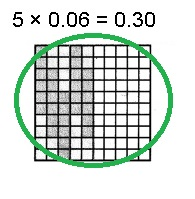Explanation:
Step 1
Making  5 groups of 6 hundredths.
Remembering that a square or a flat is equal to 1.
Drawn a quick picture to record your work.

Step 2
Combined the hundredths and rename.
There are 6 hundredths. I will rename _6__ hundredths as _0.60__.
In our quick picture, cross out the hundredths you renamed.

Step 3
Recorded the value shown by your completed quick picture
So, 5 × 0.06 = _0.30_

Question 2.
5 × 0.38 = _1.90__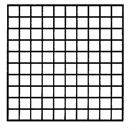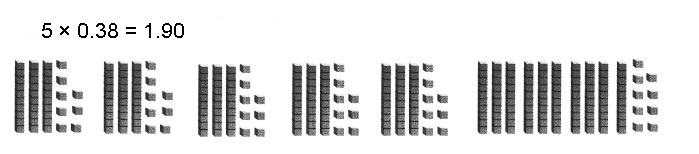Explanation:
Step 1
Making  5 groups of 3 tenths and 8  hundredths,
Remembering that a square or a flat is equal to 1.
Drawn a quick picture to record your work.

Step 2
Combined the tenths,hundredths and rename.
There are 3 tenths and 8 hundredths,
I will rename _3__ tenths and __8 __hundredths as _0.38__.
In your quick picture, cross out the hundredths you renamed.

Step 3
Recorded the value shown by your completed quick picture
So, 5 × 0.38 = _1.90_

Question 3.
4 × 0.24 = ___4 X 0.24 = 0.96,Explanation:
Step 1
Making  4 groups of 2 tenths and 4 hundredths,
Remembering that a square or a flat is equal to 1.
Drawn a quick picture to record your work.

Step 2
Combined the tenths and hundredths and rename.
There are 2 tenths, 4 hundredths. I will rename 2 tenths,
4 hundredths as _0.24__.
In your quick picture, cross out the hundredths you renamed.

Step 3
Recorded the value shown by your completed quick picture
So, 4 × 0.24 = _0.96_

Question 4.
3 × 0.62 = __1.86__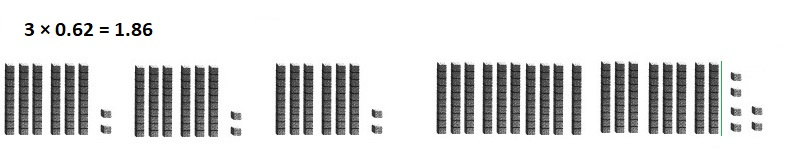Explanation:
Step 1
Making  3 groups of 6 tenths and 2 hundredths,
Remembering that a square or a flat is equal to 1.
Drawn a quick picture to record your work.

Step 2
Combined the tenths and hundredths and rename.
There are 6 tenths, 2 hundredths. I will rename 6 tenths,
2 hundredths as _0.62__.
In your quick picture, cross out the hundredths you renamed.

Step 3
Recorded the value shown by your completed quick picture
So, 3 × 0.62 = _1.86_

Question 5.
4 × 0.32 = _1.28__Explanation:
Step 1
Making 4 groups of 3 tenths and 2 hundredths,
Remembering that a square or a flat is equal to 1.
Drawn a quick picture to record your work.

Step 2
Combined the tenths and hundredths and rename.
There are 3 tenths, 2 hundredths. I will rename 3 tenths,
2 hundredths as _1.28__.
In your quick picture, cross out the hundredths you renamed.

Step 3
Recorded the value shown by your completed quick picture
So, 4 × 0.32 = _1.28_

Problem Solving

Use the table for 6-8. Use quick pictures or base-ten blocks to help.

Question 6.
Each day a bobcat drinks about 3 times as much water as a Canada goose drinks.
How much water does a bobcat drink in one day?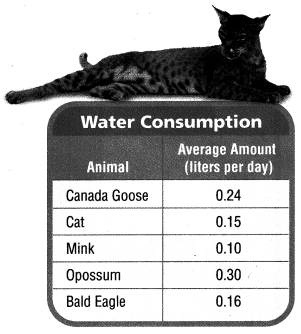3 X 0.24 = 0.72 liters,

Explanation:
Given each day a bobcat drinks about 3 times as much water as a Canada Goose drinks.
Average amount each day Canada Goose drinks is 0.24 liters,
Water does a bobcat drink in one day is
0.24
X  3
0.72

Question 7.
Multi-Step River otters drink about 5 times as much water as a bald eagle drinks in a day.
How much water does a river otter drink in three days?Water does a River otter drink in three days is 2.40 liters,

Explanation:
Given Multi-Step River otters drink about 5 times as much
water as a bald eagle drinks in a day.
Average amount each day Bald Eagle drinks is 0.16 liters
5 X 0.16 = 0.80,
Water does a River otter drink in three days is
3 X 0.80
0.80
X   3
2.40

Question 8
Write Math Explain how you could use a quick picture to
find the amount of water that a cat drinks in 5 days.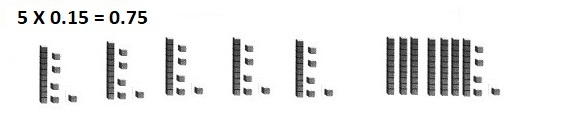The amount of water that a cat drinks in 5 days is 0.75 liters,

Explanation:
Given Math explained the amount of water that a cat drinks in
5 days is 5 X 0.15 = 0.75  liters.

Question 9.
Compare the product of 0.46 and 3 with each of the factors.
Which number has the greatest value? Explain how this is different than
multiplying two whole numbers.
The greatest values will be multiplying two whole numbers rather than
multiplying decimal values,

Explanation:
The product of 0.46 and 3 is
1
0.46
X   3
1.38
Factors of 0.4 is (0.1,0.4) or (0.2,0.2),
Factors of 0.06 is (0.01,0.06) or (0.02, 0.03),
Factors of 3 are (1,3), there are no common factors as
we have whole numbers compairing with tenths and hundredths  ,
therefore, the greatest values will be multiplying two whole numbers rather than
multiplying decimal values.

Question 10.
Representations What multiplication expression does the model show?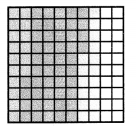(A) 5 × 0.4
(B) 5 × 0.6
(C) 16 × 0.05
(D) 5 × 0.16
(A) 5 × 0.4,

Explanation:
Given model represents 5 multiplied by 4 tenths,
So matches with (A) 5 X 0.4.

Question 11.
Display Which equation matches the model?(A) 4 × 0.31 = 1.24
(B) 4 × 0.31 = 1.51
(C) 4 × 0.39 = 1.24
(D) 4 × 0.39 = 1.56
(A) 4 × 0.31 = 1.24

Explanation:
Given model represents 4 multiplied by 3 tenths and 1 hundredths
giving the results as 4 X 0.31 =
0.31
X 4
1.24, So matches with (A) 4 X 0.31 = 1.24.

Question 12.
Multi-Step Use Diagrams Use a quick picture to multiply 3 × 0.16.
Then multiply the result by 3 again. What is the final product?
(A) 0.32
(B) 0.48
(C) 1.44
(D) 1.24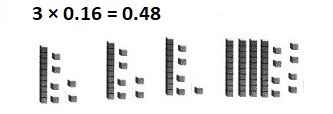(C) 1.44,

Explanation:
Using Diagrams a quick picture to multiply
0.16
X  3
0.48
Now multiplying the result by 3 again,
0.48
X  3
0.24
1.20
1.44,
matches with (C).

Texas Test Prep

Question 13.
Jared has a parakeet that weighs 1.44 ounces. Susie has a Senegal parrot that
weighs 3 times as much as Jared’s parakeet. How many ounces does Susie’s parrot weigh?
(A) 0.32 ounce
(B) 43.2 ounces
(C) 4.32 ounces
(D) 0.43 ounce
(C) 4.32 ounces,

Explanation:
Given Jared has a parakeet that weighs 1.44 ounces.
Susie has a Senegal parrot that weighs 3 times as much as Jared’s parakeet.
Number of ounces does Susie’s parrot weigh is
11
1.44
X  3
4.32 matches with (C) 4.32 ounces.

### Texas Go Math Grade 5 Lesson 3.2 Homework and Practice Answer Key

Use the decimal model to find the product.

Question 1.
4 × 0.07 = _0.28__4 X 0.07 = 0.28,

Explanation:
Given model represents 4 multiplied by 7 hundredths
giving the results as 4 X 0.07 =
0.07
X  4
0.28, So 4 X 0.07 = 0.28.

Question 2.
3 × 0.21 = __0.63_Explanation:
Given model represents 3 multiplied by 2 tenths and 1 hundredths
giving the results as 3 X 0.21 =
0.21
X  3
0.63, So 3 X 0.63 = 0.63.

Question 3.
4 × 0.13 = _0.52__Explanation:
Given model represents 4 multiplied by 1 tenths and 3 hundredths
giving the results as 4 X 0.13 =
0.13
X  4
0.52, So 4 X 0.13 = 0.52.

Find the product. Draw a quick picture.

Question 4.
3 × 0.38 = _1.14___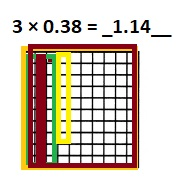Explanation:
Given model represents 3 multiplied by 3 tenths and 8 hundredths
giving the results as 3 X 0.38 =
2
0.38
X  3
1.14, So 3 X 0.38 = 1.14.

Question 5.
2 × 0.41 = __0.82__
2 X 0.41 =Explanation:
Given model represents 2 multiplied by 4 tenths and 1 hundredths
giving the results as 2 X 0.41 =
0.41
X  2
0.82, So 2 X 0.41 = 0.82.

Problem Solving

Question 6.
Lars walks his dog 4 times farther than Michelle walks her dog.
Michelle walks her dog 0.23 mile. How far does Lars walk his dog?
0.92 Lars walk his dog,

Explanation:
Lars walks his dog 4 times farther than Michelle walks her dog.
Michelle walks her dog 0.23 mile. Far does Lars walk his dog is
0.23
X  4
0.92

Question 7.
One day, Nat’s dog drank 3 times as much water as his cat.
If his cat drank 0.83 liter of water, how many liters did his dog drink?
24.9 liter Nat’s dog drank water,

Explanation:
One day, Nat’s dog drank 3 times as much water as his cat.
If his cat drank 0.83 liter of water, Liters does his dog drank is
0.83
X  3
24.9
therefore, 24.9 liter Nat’s dog drank water.

Lesson Check

Texas Test Prep

Question 8.
Which equation matches the model?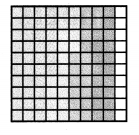(A) 5 × 0.18 = 0.9
(B) 5 × 0.18 = 90
(C) 5 × 0.18 = 9.0
(D) 5 × 0.18 = 0.09
(A) 5 × 0.18 = 0.9,

Explanation:
Given model represents 5 multiplied by 1 tenths and 8 hundredths
giving the results as 5 X 0.18 =
4
0.18
X  5
0.90, So 5 X 0.18 = 0.90.

Question 9.
Sidney’s bag of popcorn weighs 1.89 ounces. Sam’s bag of popcorn weighs
4 times as much as Sidney’s. What is the weight of Sam’s bag of popcorn?
(A) 0.756 ounce
(B) 7.56 ounces
(C) 75.6 ounces
(D) 756 ounces
(B) 7.56 ounces,

Explanation:
Given Sidney’s bag of popcorn weighs 1.89 ounces. Sam’s bag of popcorn weighs
4 times as much as Sidney’s. So the weight of Sam’s bag of popcorn is
33
1.89
X  4
7.56
ounces which matches with (B).

Question 10.
A beaker is filled with 0.23 liter of water. Leon fills another beaker with
3 times as much water. How much water is in Leon’s beaker?
(A) 0.96 liter
(B) 0.84 liter
(C) 0.69 liter
(D) 0.47 liter
(C) 0.69 liter,

Explanation:
Given a beaker is filled with 0.23 liter of water. Leon fills another beaker with
3 times as much water. So water is in Leon’s beaker is
0.23
X  3
0.69 liter
which matches with (C).

Question 11.
In a swimming race, Yvonne swam 0.18 mile. David swam
4 times as far as Yvonne. How far did David swim?
(A) 2.2 miles
(B) 0.22 mile
(C) 7.2 miles
(D) 0.72 mile
(D) 0.72 mile,

Explanation:
Given in a swimming race, Yvonne swam 0.18 mile. David swam
4 times as far as Yvonne. So far did David swim is
3
0.18
X  4
0.72 mile matches with (D).

Question 12.
Multi-Step Carrie has 0.73 liters of juice in her pitcher.
Sanji’s pitcher has 2 times as much juice as Carrie’s pitcher.
Lee’s pitcher has 4 times as much juice as Carrie’s pitcher.
Sanji and Lee pour all their juice into a large bowl. How much juice is in the bowl?
(A) 1.46 liters
(B) 4.38 liters
(C) 2.92 liters
(D) 5.11 liters
(B) 4.38 liters,

Explanation:
Given Multi-Step Carrie has 0.73 liters of juice in her pitcher.
Sanji’s pitcher has 2 times as much juice as Carrie’s pitcher.
So Sanji’s pitcher has 2 X 0.73 = 1.46 liters,
Lee’s pitcher has 4 times as much juice as Carrie’s pitcher.
So Lee’s pitcher has 4 X 0.73 = 2.92 liters,
As Sanji and Lee pour all their juice into a large bowl.
Juice is in the bowl is 1.46 + 2.92 = 4.38 liters matches with (B).

Question 13.
Multi-Step Justin and Hayden are playing a number game.
Justin’s number is the product of 4 × 0.06. Hayden’s number 0.04
less than the product of Justin’s number and 4. What is Hayden’s number?
(A) 0.92
(B) 0.96
(C) 0.60
(D) 1.0